[sān jiǎo hán shù]

# 三角函数

## （数学名词）

trigonometric function

## 三角函数发展历史

### 三角函数阿拉伯历史

18世纪开始，随着解析几何等分析学工具的引进，数学家们开始对三角函数进行分析学上的研究。牛顿在1669年的《分析学》一书中给出了正弦和余弦函数的无穷级数表示。Collins将牛顿的结果告诉了詹姆斯·格列高里，后者进一步给出了正切等三角函数的无穷级数。莱布尼兹在1673年左右也独立得到了这一结果。欧拉的《无穷小量分析引论》（Introductio in Analysin Infinitorum，1748年）对建立三角函数的分析处理做了最主要的贡献，他定义三角函数为无穷级数，并表述了欧拉公式，还有使用接近现代的简写sin.cos.tang.cot.sec.cosec.

## 三角函数定义

### 三角函数直角三角形三角函数定义

 基本函数 英文 缩写 表达式 语言描述 正弦函数 sine sin a/c ∠A的对边比斜边 余弦函数 cosine cos b/c ∠A的邻边比斜边 正切函数 tangent tan a/b ∠A的对边比邻边 余切函数 cotangent cot b/a ∠A的邻边比对边 正割函数 secant sec c/b ∠A的斜边比邻边 余割函数 cosecant csc c/a ∠A的斜边比对边

### 三角函数基本三角函数关系的速记方法

1）对角相乘乘积为1，即sinθ·cscθ=1； cosθ·secθ=1； tanθ·cotθ=1。
2）六边形任意相邻的三个顶点代表的三角函数，处于中间位置的函数值等于与它相邻两个函数值的乘积，如：sinθ=cosθ·tanθ；tanθ=sinθ·secθ...
3）阴影部分的三角形，处于上方两个顶点的平方之和等于下顶点的平方值，如：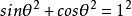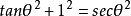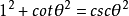### 三角函数变化规律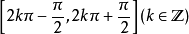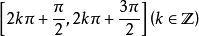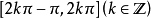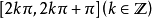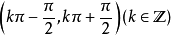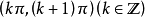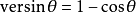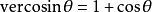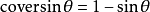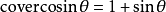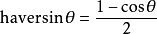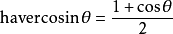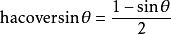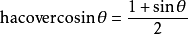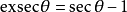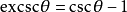### 三角函数任意角三角函数定义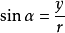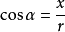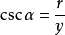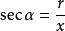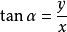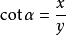### 三角函数级数定义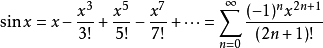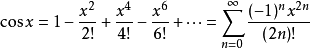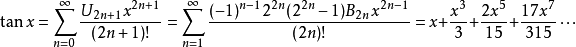## 三角函数三角学

1464年，他以雷基奥蒙坦纳斯的名字发表了《各种三角形》。在书中，他把以往散见在各种书上的三角学知识，系统地综合了起来，成了三角学在数学上的一个分支，

## 三角函数特殊角

 角度 0° 15° 30° 45° 60° 90° 120° 135° 150° 180° 270° 弧度 0 π/12 π/6 π/4 π/3 π/2 2π/3 3π/4 5π/6 π 3π/2 sin值 0 [（√6）-（√2）]/4 1/2 （√2）/2 （√3）/2 1 （√3）/2 （√2）/2 1/2 0 -1 cos值 1 [（√6）+（√2）]/4 （√3）/2 （√2）/2 1/2 0 -1/2 -（√2）/2 -（√3）/2 -1 0 tan值 0 2-√3 （√3）/3 1 √3 ∅ -√3 -1 -（√3）/3 0 ∅ cot值 ∅ 2+√3 √3 1 √3）/3 0 -（√3）/3 -1 -√3 ∅ 0

## 三角函数几何性质

y=sin x

x=kπ+π/2（k∈Z）
（kπ,0）（k∈Z）
y=cos x

x=kπ（k∈Z）
（kπ+π/2,0）（k∈Z）
y=tan x

kπ/2+π/2,0）（k∈Z）
y=cot x

kπ/2,0）（k∈Z）
y=sec x

x=kπ（k∈Z）
（kπ+π/2,0）（k∈Z）
y=csc x

x=kπ+π/2（k∈Z）
（kπ,0）（k∈Z）

### 三角函数最小正周期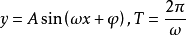## 三角函数诱导公式

### 三角函数公式内容

 公式一 公式二 sin（2kπ+α）=sin α cos（2kπ+α）=cos α tan（2kπ+α）=tan α cot（2kπ+α）=cot α sec（2kπ+α）=sec α csc（2kπ+α）=csc α sin（π+α）=-sin α cos（π+α）=-cos α tan（π+α）=tan α cot（π+α）=cot α sec（π+α）=-sec α csc（π+α）=-csc α 公式三 公式四 sin（-α）=-sin α cos（-α）=cos α tan（-α）=-tan α cot（-α）=-cot α sec（-α）=sec α csc（-α）=-csc α sin（π-α）=sin α cos（π-α）=-cos α tan（π-α）=-tan α cot（π-α）=-cot α sec（π-α）=-sec α csc（π-α）=csc α 公式五 公式六 sin（α-π）=-sin α cos（α-π）=-cos α tan（α-π）=tan α cot（α-π）=cot α sec（α-π）=-sec α csc（α-π）=-csc α sin（2π-α）=-sin α cos（2π-α）=cos α tan（2π-α）=-tan α cot（2π-α）=-cot α sec（2π-α）=sec α csc（2π-α）=-csc α 公式七 公式八 sin（π/2+α）=cosα cos（π/2+α）=−sinα tan（π/2+α）=-cotα cot（π/2+α）=-tanα sec（π/2+α）=-cscα csc（π/2+α）=secα sin（π/2-α）=cosα cos（π/2-α）=sinα tan（π/2-α）=cotα cot（π/2-α）=tanα sec（π/2-α）=cscα csc（π/2-α）=secα 公式九 公式十 sin（3π/2+α）=-cosα cos（3π/2+α）=sinα tan（3π/2+α）=-cotα cot（3π/2+α）=-tanα sec（3π/2+α）=cscα csc（3π/2+α）=-secα sin（3π/2-α）=-cosα cos（3π/2-α）=-sinα tan（3π/2-α）=cotα cot（3π/2-α）=tanα sec（3π/2-α）=-cscα csc（3π/2-α）=-secα

### 三角函数推导方法

90°的奇数倍+α的三角函数，其绝对值与α三角函数的绝对值互为余函数。90°的偶数倍+α的三角函数与α的三角函数绝对值相同。也就是“奇余偶同，奇变偶不变”。

## 三角函数关于三角恒等式

### 三角函数两角和与差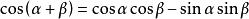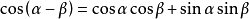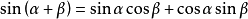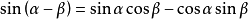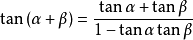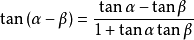∵A（cosα,sinα），B （cosβ,sinβ），O（0,0）
OA=（cosα,sinα），OB=（cosβ,sinβ）（向量
∴OA·OB=|OA| |OB| cos （α-β） =cos α cos β + sin α sin β
∵|OA| = |OB| = 1
∴cos（α-β）=cosαcosβ+sinαsinβ

### 三角函数和差化积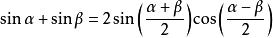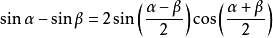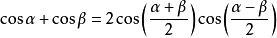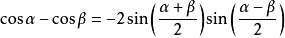### 三角函数积化和差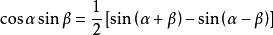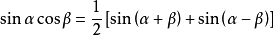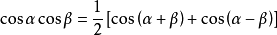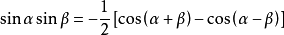### 三角函数二倍角公式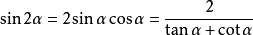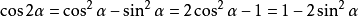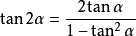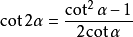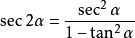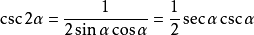### 三角函数三倍角公式

sin（3α） = 3sinα-4sin3α = 4sinα·sin（60°+α）sin（60°-α）
cos（3α） = 4cos3α-3cosα = 4cosα·cos（60°+α）cos（60°-α）
tan（3α） = （3tanα-tan3α）/（1-3tan²α） = tanαtan（π/3+α）tan（π/3-α）
cot（3α）=（cot3α-3cotα）/（3cot2α-1）

### 三角函数n倍角公式

sin（nα）=ncosn-1α·sinα-C（n,3）cosn-3α·sin3α+C（n,5）cosn-5α·sin5α-…
cos（nα）=cosnα-C（n,2）cosn-2α·sin2α+C（n,4）cosn-4α·sin4α

### 三角函数半角公式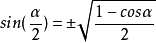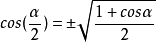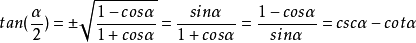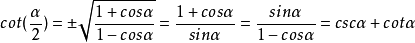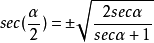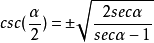### 三角函数辅助角公式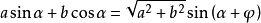（其中φ满足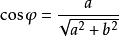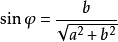### 三角函数万能公式

sina=[2tan（a/2）]/[1+tan²（a/2）]
cosa=[1-tan²（a/2）]/[1+tan²（a/2）]
tana=[2tan（a/2）]/[1-tan²（a/2）]

### 三角函数降幂公式

sin²α=[1-cos（2α）]/2
cos²α=[1+cos（2α）]/2
tan²α=[1-cos（2α）]/[1+cos（2α）]

### 三角函数三角和

sin（α+β+γ）=sinα·cosβ·cosγ+cosα·sinβ·cosγ+cosα·cosβ·sinγ-sinα·sinβ·sinγ
cos（α+β+γ）=cosα·cosβ·cosγ-cosα·sinβ·sinγ-sinα·cosβ·sinγ-sinα·sinβ·cosγ
tan（α+β+γ）=（tanα+tanβ+tanγ-tanα·tanβ·tanγ）÷（1-tanα·tanβ-tanβ·tanγ-tanγ·tanα）

### 三角函数幂级数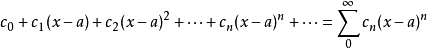### 三角函数泰勒展开式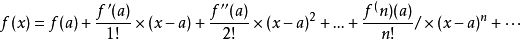ex = 1+x+x2/2!+x3/3!+…+xn/n!+…,x∈R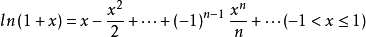sin x = x-x3/3!+x5/5!-…+（-1）k-1x2k-1/（2k-1）!+…, x∈R
cos x = 1-x2/2!+x4/4!-…+（-1）kx2k/（2k）!+…, x∈R
arcsin x = x + x3/（2×3） + （1×3）x5/（2×4×5） + （1×3×5）x7/（2×4×6×7）…+（2k-1）!!×x2k+1/（2k!!×（2k+1））+…, x∈（-1,1）（!!表示双阶乘）
arccos x = π/2 -[x + x3/（2×3） + （1×3）x5/（2×4×5） + （1×3×5）x7/（2×4×6×7）……], x∈（-1,1）
arctan x = x - x3/3 + x5/5 -…, x∈（-∞,1）
sinh x = x+x3/3!+x^5/5!+…+x2k-1/（2k-1）!+…, x∈R
cosh x = 1+x2/2!+x^4/4!+…+x2k/（2k）!+…, x∈R
arcsinh x =x - x3/（2×3） + （1×3）x5/（2×4×5） -（1×3×5）x7/（2×4×6×7）…, x∈（-1,1）
arctanh x = x + x3/3 + x5/5 + …, x∈（-1,1）

### 三角函数傅里叶级数

f（x）=a0/2+∑（n=0..∞） （ancosnx+bnsinnx）
a0=1/π∫（π..-π） （f（x））dx
an=1/π∫（π..-π） （f（x）cosnx）dx
bn=1/π∫（π..-π） （f（x）sinnx）dx

## 三角函数概念

sin（x），cos（x）的定义域为R，值域为[-1,1]。
tan（x）的定义域为x不等于π/2+kπ（k∈Z），值域为R
cot（x）的定义域为x不等于kπ（k∈Z）,值域为R
y=a·sin（x）+b·cos（x）+c 的值域为 [ c-√（a&sup2；+b&sup2；） , c+√（a&sup2；+b&sup2；）]

y=sinx→【左移（φ>0）/右移（φ<0） ∣∣∣φ∣个单位】 →y=sin（x+φ）→【纵坐标不变，横坐标伸缩到原来的（1/ω）】→y=sin（ωx+φ）

y=sinx→【纵坐标不变，横坐标伸缩到原来的（1/ω）】→y=sinωx→【左移（φ>0）/右移（φ<0）∣φ∣/ω 个单位】→y=sin（ωx+φ） →【纵坐标变为原来的A倍（伸长[A>1] / 缩短[0<A<1]）】→ y=Asin（ωx+φ）

 y=sinx y'=cosx y=cosx y'=-sinx y=tanx y'=1/cos²x =sec²x y=cotx y'= -1/sin²x= - csc²x y=secx y'=secxtanx y=cscx y'=-cscxcotx y=arcsinx y'=1/√（1-x²） y=arccosx y'= -1/√（1-x²） y=arctanx y'=1/（1+x²） y=arccotx y'= -1/（1+x²）

y=arcsin（x），定义域[-1,1]，值域[-π/2,π/2]，图象用红色线条；
y=arccos（x），定义域[-1,1]，值域[0,π]，图象用蓝色线条；
y=arctan（x），定义域（-∞，+∞），值域（-π/2,π/2），图象用绿色线条；
sinarcsin（x）=x,定义域[-1,1],值域 [-π/2,π/2]

## 三角函数推广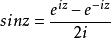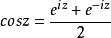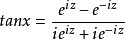·三角函数作为微分方程的解：

Q=Asinx+Bcosx，因此也可以从此出发定义三角函数。

## 三角函数复数性质

（1）对于z为实数y来说，复数域内正余弦函数的性质与通常所说的正余弦函数性质是一样的。
（2）复数域内正余弦函数在z平面是解析的。
（3）在复数域内不能再断言|sinz|≦1,|cosz|≦1。
（4）sinz、cosz分别为奇函数偶函数，且以2π为周期。

sin（a+bi）=sinacosbi+sinbicosa
=sinachb+ishbcosa
cos（a-bi）=cosacosbi+sinbisina
=cosachb+ishbsina
tan（a+bi）=sin（a+bi）/cos（a+bi）
cot（a+bi）=cos（a+bi）/sin（a+bi）
sec（a+bi）=1/cos（a+bi）
csc（a+bi）=1/sin（a+bi）

## 三角函数相关定理

### 三角函数正弦定理

sinA / a = sinB / b = sinC/c

a/sinA=b/sinB=c/sinC=2R

S=1/2absinC=1/2bcsinA=1/2acsinB

### 三角函数余弦定理

a² = b² + c²- 2bc·cosA
b² = a² + c² - 2ac·cosB
c² = a² + b² - 2ab·cosC

cosC=（a² +b² －c²）/ 2ab
cosB=（a² +c² -b²）/ 2ac
cosA=（c² +b² -a²）/ 2bc

a=b·cos C+c·cos B， b=c·cos A+a·cos C， c=a·cos B+b·cos A

### 三角函数正切定理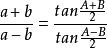### 三角函数三角恒等式

tanA+tanB+tanC=tanAtanBtanC

## 三角函数记忆口诀

• 1.    莫里斯·克莱因 著，张理京，张锦炎，江泽涵 译. 《古今数学思想》第一册. 上海科学技术出版社. 2002.
• 2.    R. P. Brent, "Fast Multiple-Precision Evaluation of Elementary Functions", J. ACM 23, 242 (1976).